### 3. POLARIZATION FROM THOMSON SCATTERING

Polarization in the microwave background is generated through the polarization-dependent cross-section for Thomson scattering. Consider Thomson scattering of an incoming unpolarized beam of electromagnetic radiation by an electron; this discussion closely follows those in Kosowsky (1996) and Kosowsky (1998). The total scattering cross-section, defined as the radiated intensity per unit solid angle divided by the incoming intensity per unit area, is given by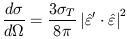(9)

whereT is the total Thomson cross section and the vectors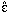and' are unit vectors in the planes perpendicular to the propogation directions which are aligned with the outgoing and incoming polarization, respectively. Consider first a nearly monochromatic, unpolarized incident plane wave of intensity I' and cross-sectional areaB which is scattered into the z-axis direction. Defining the y-axes of the incoming and outgoing coordinate systems to be in the scattering plane, the Stokes parameters of the outgoing beam, defined with respect to the x-axis, follow from Eq. (9) as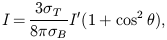(10)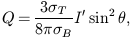(11)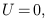(12)

whereis the angle between the incoming and outgoing beams. By symmetry, Thomson scattering can generate no circular polarization, so V = 0 always and will not be considered further. (Note that Eqs. (3) give the well-known result that sunlight from the horizon at midday is linearly polarized parallel to the horizon).

The net polarization produced by the scattering of an incoming, unpolarized ratiation field of intensity I'(,) is determined by integrating Eqs. (3) over all incoming directions. Note that the coordinate system for each incoming direction must be rotated about the z-axis so that the outgoing Stokes parameters are all defined with respect to a common coordinate system, using the transformation of Q and U under rotations. The result is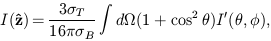(13)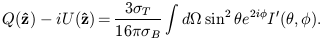(14)

Expanding the incident radiation field in spherical harmonics,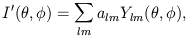(15)

leads to the following expressions for the outgoing Stokes parameters: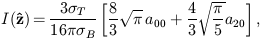(16)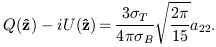(17)

Thus polarization is generated along the outgoing z-axis provided that the a22 quadrupole moment of the incoming radiation is non-zero. To determine the outgoing polarization in a direction making an anglewith the z-axis, the same physical incoming field must be multipole expandedin a coordinate system rotated through the Euler angle; the rotated multipole coefficients are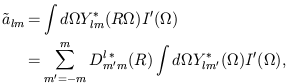(18)

where R is the rotation operator and Dlm'm is the Wigner D-symbol. (For a wonderfully complete reference on representations of the rotation group, see Varshalovich et al. (1988)). In the rotated coordinate system, the multipole coefficient generating polarization is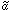22 by Eqs. (3). The unrotated multipole components which contribute to polarization will all have l = 2 by the orthogonality of the spherical harmonics. If the incoming radiation field is independent of, as it will be for individual Fourier components of a density perturbation, then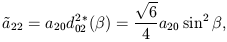(19)

which has used an explicit expression for the reduced D-symbol dlm'm. The outgoing Stokes parameters are finally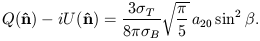(20)

In other words, an azimuthally-symmetric radiation field will generate a polarized scattered field if it has a non-zero a20 multipole component, and the magnitude of the scattered polarization will be proportional to sin2. Since the incoming field is real, a20 will be real, U = 0, and the polarization orientation will be in the plane of the z-axis and the scattering direction. Similar relationships can be derived for radiation fields which are not azimuthally symmetric, which occur in the cases of vector and tensor metric perturbations.

In short, what this section shows is that unpolarized quadrupolar radiation fields get Thomson scattered into polarized radiation fields. This is the key fact which must be appreciated to understand why the microwave background should be polarized and what the magnitude of the polarization is expected to be.